Select PageGrade 7 is a very important year. Ezio therefore wrote a Explanations and Workbook with integrated papers. Algebra and Finances are new concepts. The book consists of 332 pages. The book has a complete Answer Sheet.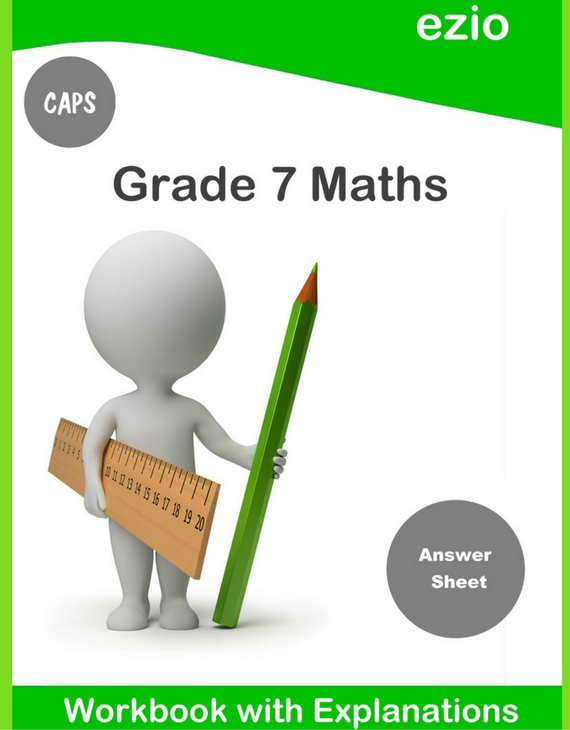Ezio Grade 7 Workbook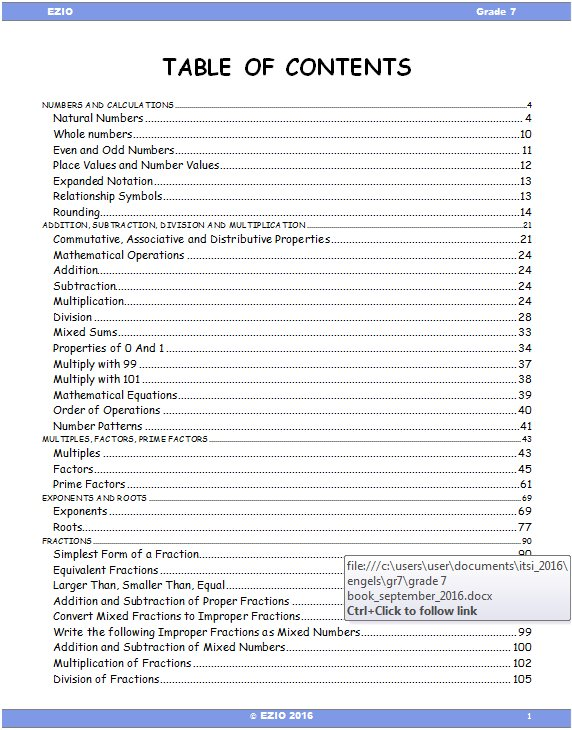Numbers And Calculations
Addition, Subtraction, Division And Multiplication
Multiples, Factors, Prime Factors
Exponents And Roots
Fractions
Decimals
Percentage
Money
Ratio, Rate And Proportion
Algebra
Integers
Geometry And Construction
2d And 3d Shapes
Perimeter, Area And Volume
Statistics, Graphs, Data Handling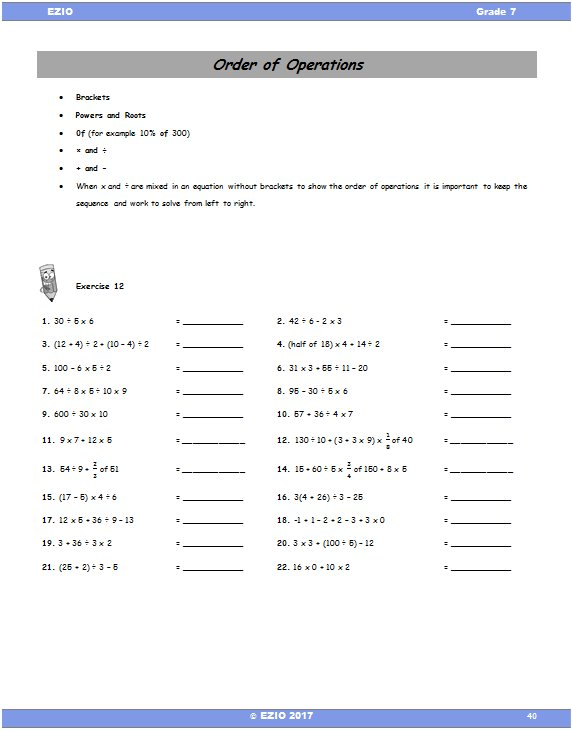Example
Order of Operations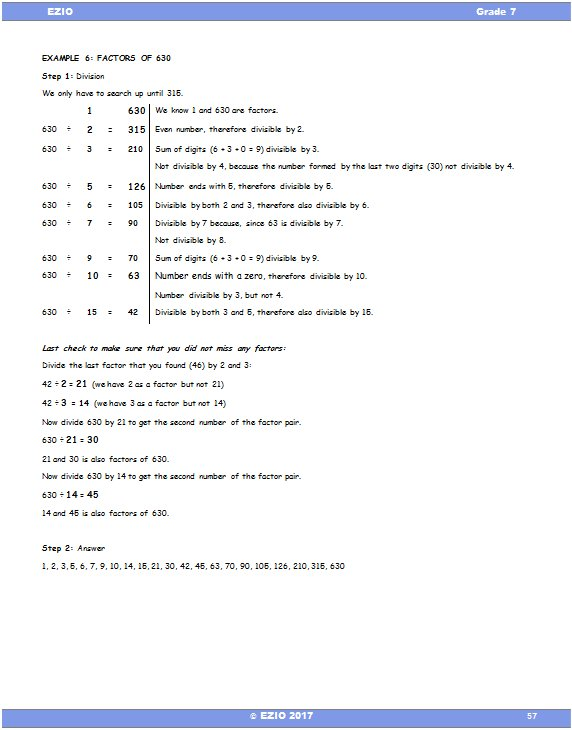Example
Factors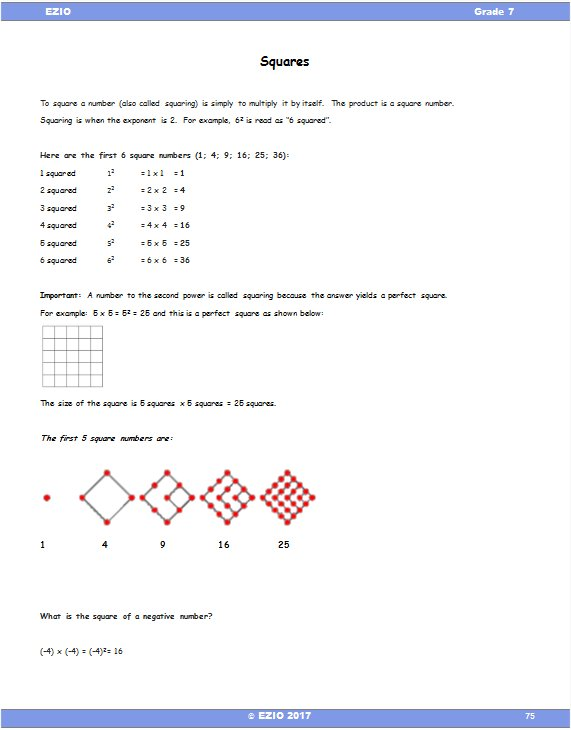Example
Squares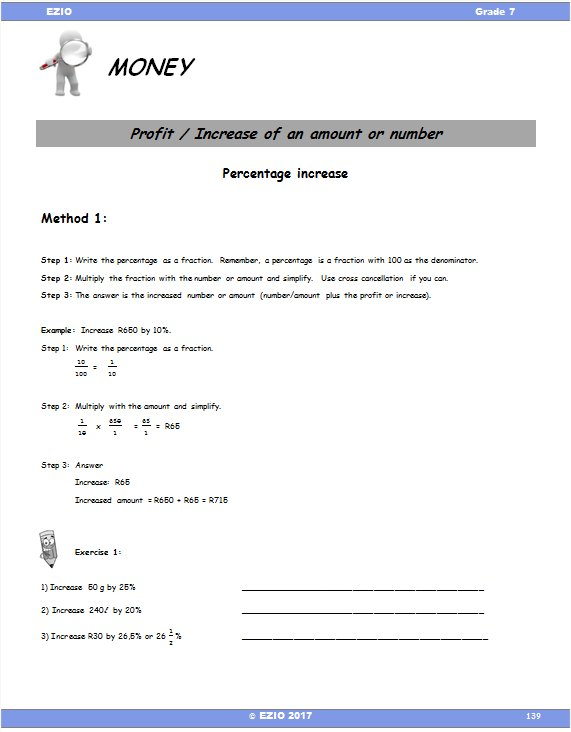Example
Money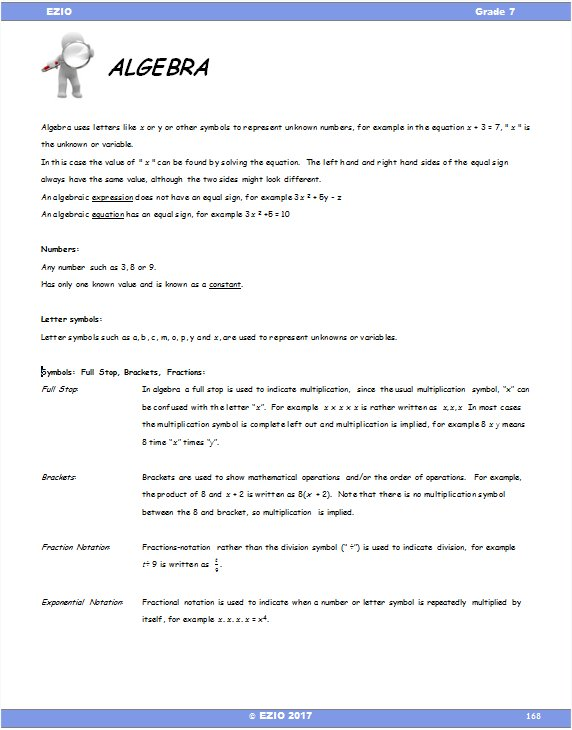Example
Algebra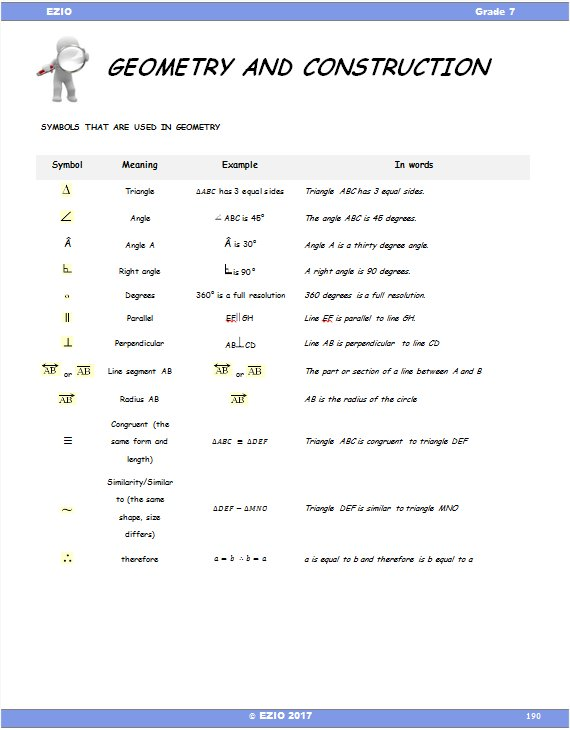Example
Geometry and Construction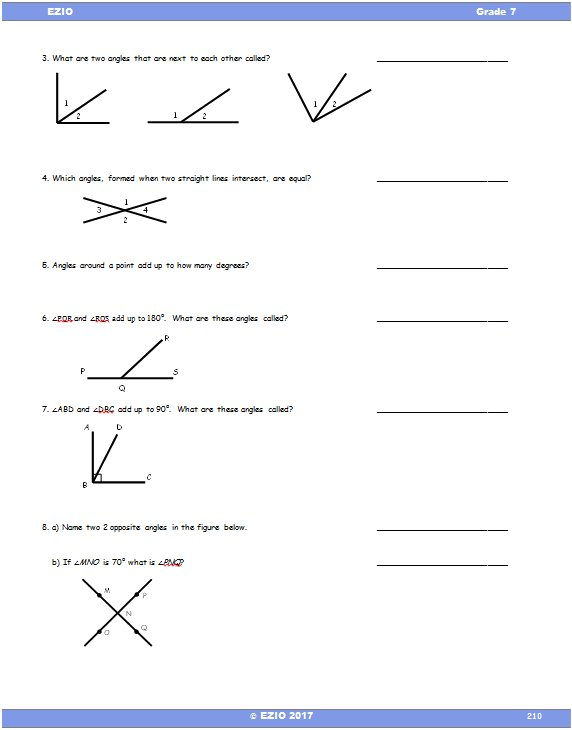Example
Angles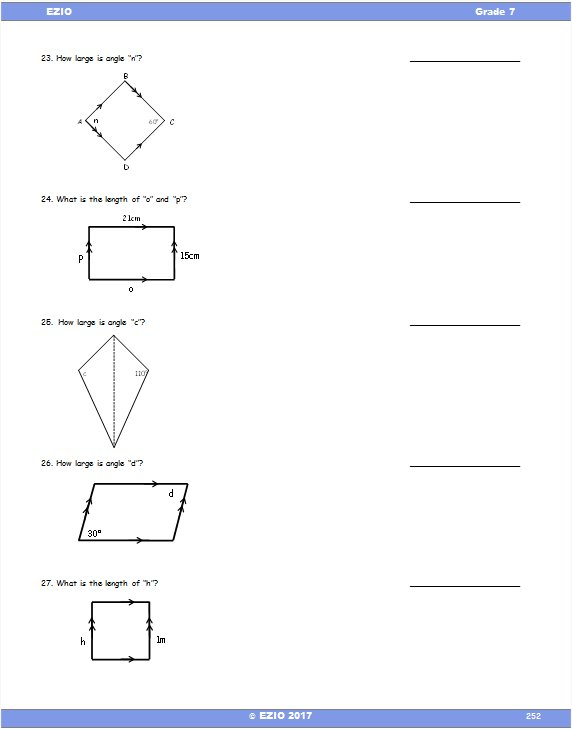Example
Angles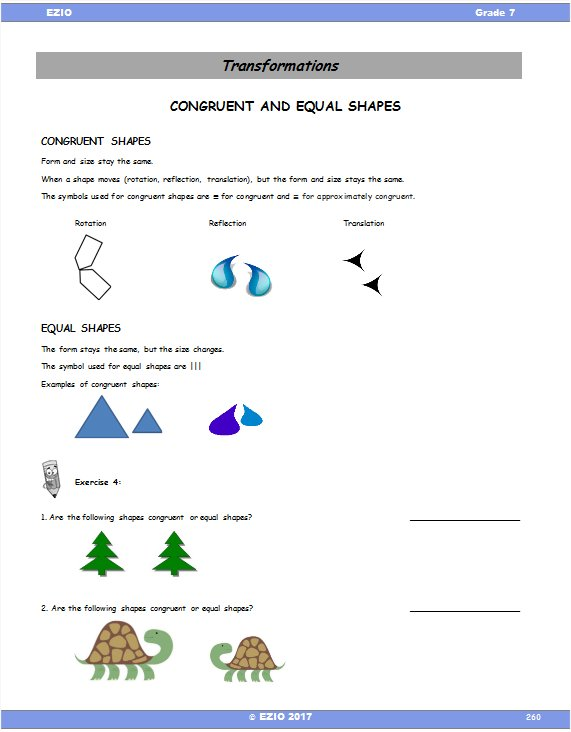Example
Transformations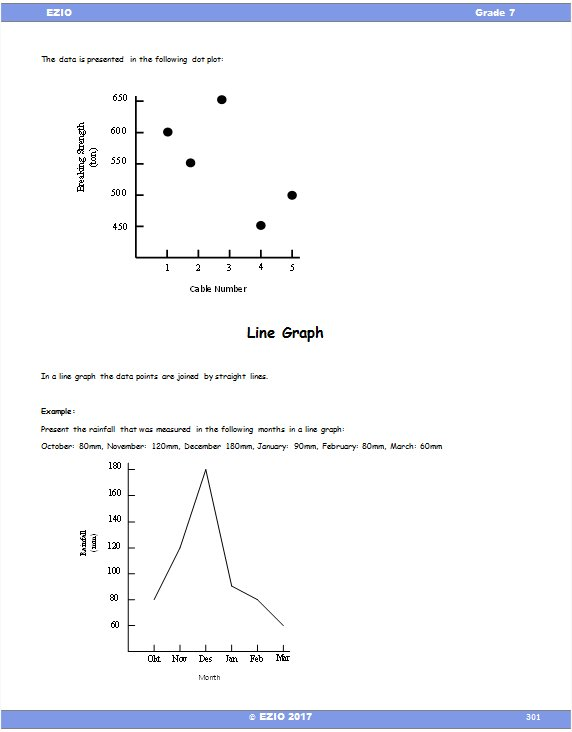Example
Graphs